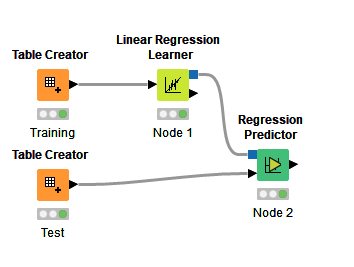# How to get the Coefficient and Intercept from Linear regression learner to do math calculation

Hi there,
I have a question:
I have two data sets: the first data set are my training data, which can be used to generate a Linear regression between two parameters (y = ax + b), and I want to use the a and b from this equation to calculate the unknown Y with known X with the same equation: Y = aX + b.
The linear regression node seems can get the a and b (coefficient and intercept), but I don’t know how to use them to do the next step calculation for my second dataset.

Do you have any advice?

You should familiarize yourself with the Learner-Predictor construct: https://www.knime.com/knime-introductory-course/chapter6/section1/learner-predictor-construct

Connect the blue model output port of the Linear Regression Learner node to the blue model input port of the Regression Predictor node.

Since you already have split data sets, your test data will go straight into the data input port of the Regression Predictor node.4 Likes